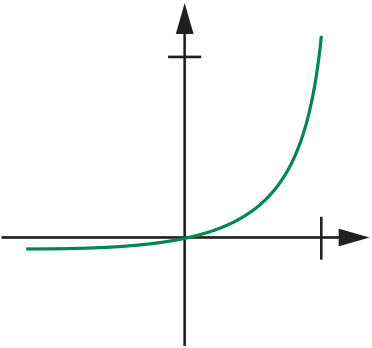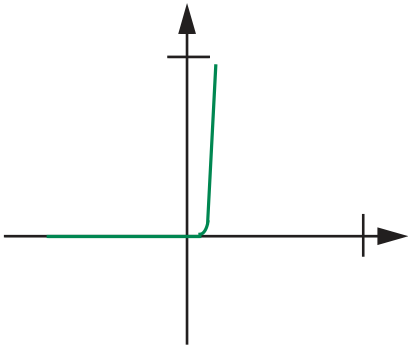Rating : ⭐⭐⭐⭐⭐
Price : \$10.99
Language:EN
Pages: 2

# This called piecewise linear analysis206 C H A P T E R

F O U R

o f n o n l i n e a r

Second, in contrast to all previous examples, the output waveform in this circuit is a gross distortion of the input waveform. Note in particular that the input voltage waveform has no average value, (no DC value), whereas the output has a significant DC component, roughly 0.3 Eo. The DC motors in most toys, for example, will run nicely if connected across the resistor in the circuit of Figure 4.21a, whereas they will not run if driven directly by the sinusoid vI(t). This circuit is called a half-wave rectifier, because it reproduces only half of the input wave. Rectifiers are present in power supplies of most electronic equipment to generate DC from the 60-Hz ‘‘sinusoidal’’ wave from 110-V AC power line.

4.4

10 pA

iD

10 mA

iD

different scales. (a) 0.5 V
(b) 5 V
4.4 Piecewise Linear Analysis

C H A P T E R

F O U R

207

circuit

crudest approximation that preserves this dichotomy is the characteristic shown

vD
Open

(a)

+

The behavior of this piecewise linear model can be summarized in two

circuit

illustrate the piecewise linear analysis method applied to the circuit in Figure 4.16

iD

(also shown in Figure 4.24a). In particular, we will determine the voltage vR

vD

+
E
-

(a)

+ vR -
+
+ vR
iD

+

FIGURE 4.23 A piecewise

R R
+
vD
- (b) Short circuit segment

-

FIGURE 4.24 Piecewise linear
vR
analysis of a simple diode circuit.
iD
R
+
vD

-

How It Works